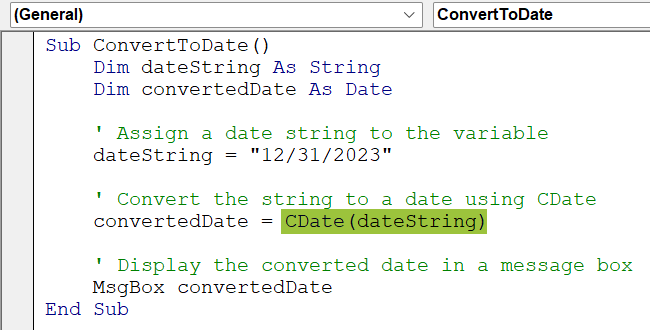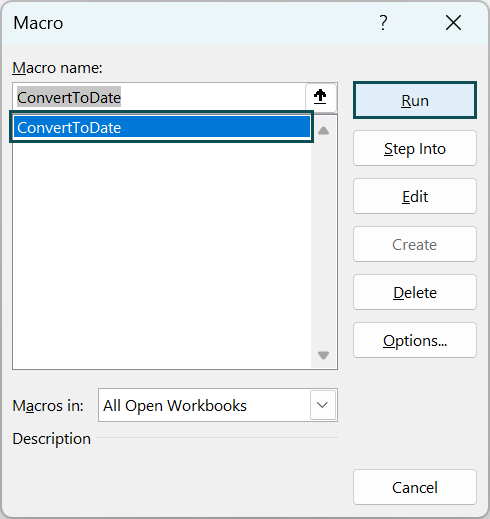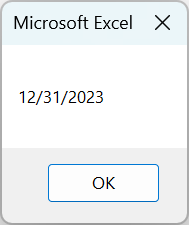# VBA CDATE FunctionArticle byExcelMojo Team## What is CDATE Function in VBA?

The VBA CDATE function is a tool for converting expressions into “Date” data types. It stands for “Convert to Date.” This function converts strings, numeric values, and objects into valid date representations.

CDATE is particularly useful when working with dates in VBA, as it simplifies converting different data types into a standardized date format.

We used the VBA CDATE Function in the above example to convert a numeric date into the “dd-mm-yyyy” format.

Note: A numeric date is a date representation as a numeric value, typically a serial number, used to represent dates in certain applications. In Excel, numeric dates are based on the number of days that have passed since January 1, 1900 (for Windows systems) or January 1, 1904 (for Mac systems). Numeric dates are often used for calculations or date manipulation, allowing mathematical operations and comparisons to be performed easily.

###### Key Takeaways
• VBA CDATE Function converts an expression into a Date data type.
• The syntax of the VBA CDATE Function is “CDATE(expression).”
• The VBA CDATE Function can convert strings, numeric values, and objects into valid date representations.
• To use VBA CDATE Function in Excel, open the Visual Basic Editor, write the code using the function, and assign the converted value to a variable.

### Syntax of CDATE

The syntax of the VBA CDATE function in VBA is straightforward.

“CDATE(expression)”

The “expression” parameter represents the value that you want to convert into a Date data type. It can be a string, a numeric value, or an object interpreted as a date. The VBA CDATE Function takes this expression and attempts to convert it into a valid date representation.

### How to Use the CDATE Function in Excel VBA?

To use the VBA CDATE Function in Excel, follow these steps:

1. Open the Visual Basic Editor:

Press “Alt + F11” in Excel to open the Visual Basic for Applications (VBA) editor.

2. Insert a new module:

In the VBA editor, click “Insert” in the top menu and choose “Module” to insert a new code module.

3. Copy the following code (Sub ConvertToDate.) and paste it into the newly created module in the VBA editor.

Sub ConvertToDate()
Dim dateString As String
Dim convertedDate As Date
‘ Assign a date string to the variable
dateString = “12/31/2023”
‘ Convert the string to a date using CDate
convertedDate = CDate(dateString)
‘ Display the converted date in a message box
MsgBox convertedDate
End Sub4. Modify the date string:

By default, the code uses the date string “12/31/2023.” If you want to convert a different date, modify the value assigned to the “dateString” variable. Ensure the date string follows a recognizable format (e.g., “mm/dd/yyyy”).

5. Run the code:

Close the VBA editor and return to the Excel workbook. Press “Alt + F8” to open the macro dialog box. Select the “ConvertToDate” macro from the list and click “Run” to execute the code.6. View the result:

After running the code, a message box displaying the converted date will appear. In this example, the message box will show “12/31/2023” as the corrected date.The following is a step-by-step guide on effectively using the CDATE function in Excel VBA.

1. Syntax: The VBA CDATE Function has the syntax “CDATE(expression)”. The expression is the value to be converted into a date. Ensure you understand the syntax before using the function.
1. Assign Converted Date: Declare a Date variable and assign the converted date using the CDATE function. For example, “myDate = CDATE(“2023-06-08″).” It assigns the converted date to the variable “myDate.”
1. Handle Invalid Dates: The CDATE function may raise an error if the input cannot be interpreted as a valid date. Use error-handling techniques or validate the input using the IsDate function before using CDATE.
1. Format the Result: Customize the date format using the Format function. Specify the desired format, such as “dd-mm-yyyy” or “mm/dd/yyyy.” Apply the Format function to the converted date to achieve the desired formatting.
1. Use the Converted Date: Once the value is converted and formatted, you can use the converted date in your VBA code. Perform calculations and comparisons, or use built-in VBA functions and operators with the converted date.

### Examples

To understand how to use VBA CDATE, let us look at some interesting examples, as shown below.

#### Example #1

In this example, we will use VBA CDATE Function, which converts a specified date (“15/09/1995”) to a string representation in the format “dd month yyyy” and displays it in a message box.

• Step 1: Open the Visual Basic Editor (VBE)

To open the VBE, press the Alt + F11 keys in Excel or click on the Developer tab in the Excel ribbon and then click the Visual Basic button.

• Step 2: Create a new module by clicking on the “Insert” menu and selecting “Module.” Now, create a new Subroutine. We start by declaring a subroutine named DisplayDateInWords. Subroutines are blocks of code that perform specific tasks.
• Step 3: Variable Declarations

We declare two variables: inputDate of type Date to store the input date and dateString of type String to store the formatted date in words.

• Step 4: Set the Input Date.

We set the value of inputDate to a specific date. You can modify this line to dynamically take the date input from the user or a cell in Excel.

• Step 5: Convert Date to String in Words Format

We use the Format function to convert the inputDate to a string representation with the desired format. In this case, the format pattern “dd mmmm yyyy” specifies that the date should be displayed with the day, full month name, and the four-digit year.

• Step 6: Display the Date in a Message Box.

We display the dateString in a message box using the MsgBox function. The message box will show the formatted date in words, such as “15 September 1995”.

• Step 7: Now save the code and close the VBE and press Alt + F8 and you can run the “DisplayDateInWords” VBA code.
• Step 8: Once you execute the code, you will see the message box displaying the date in the word format.

Here is the full code:

Sub DisplayDateInWords()
Dim inputDate As Date
Dim dateString As String
‘ Set the input date
inputDate = DateValue(“15/09/1995”)
‘ Convert the date to a string in words format
dateString = Format(inputDate, “dd mmmm yyyy”)
‘ Display the date in a message box
MsgBox dateString
End Sub

#### Example #2

The code example showcases a VBA function, ConvertDateToLongFormat, that takes a date string as input and converts it into a long date format, providing a formatted representation of the date in the form “Weekday, Month Day, Year.”

• Step 1: In the new module, start by declaring a Function.

The code begins by declaring a function named “ConvertDateToLongFormat.” It takes a single parameter, “dateString” of type String, representing the date in a specific format.

• Step 2: Variable Declaration

A variable named “convertedDate” is declared of type Date. This variable will store the converted date value.

• Step 3: The “DateValue” function converts the “dateString” into a Date data type. It interprets the string and converts it into a valid date value. The converted date is then assigned to the “convertedDate” variable.
• Step 4: Format the Date and end Function

The Format function is utilized to format the “convertedDate” value into the desired long date format.

In this example, the format is “dddd, mmmm d, yyyy,” which displays the date in “Weekday, Month Day, Year.” The formatted date is then assigned as the return value of the function.

The “ConvertDateToLongFormat” function takes a string representing a date, converts it into a valid Date value using the “DateValue” function, formats the date using the Format function, and returns the formatted long date as a string.

• Step 5: Save the module and exit the VBE.
• Step 6: To convert a date into the long date format in an Excel worksheet, you can utilize the “ConvertDateToLongFormat” function.

Enter the formula “=ConvertDateToLongFormat(Cell)” in any cell, replacing “Cell” with the cell reference containing the short date.

For instance, if the short date is located in cell A2, the formula would be “=ConvertDateToLongFormat(A2)”.

• Step 7: Once you enter the formula and press enter, you will notice that Cell B2 will show the long date.

Here is the full code:

Function ConvertDateToLongFormat(dateString As String) As String
Dim convertedDate As Date
convertedDate = DateValue(dateString)
ConvertDateToLongFormat = Format(convertedDate, “dddd, mmmm d, yyyy”)
End Function

#### Example #3

In this example, we will leverage the “ConvertDateToLongFormat” function mentioned in the earlier example and create a subroutine that converts all the dates in column A of an Excel worksheet.

The converted long dates will be displayed in column B.

Once we end the function declaration, we will continue in the same module to create “ConvertDatesInColumn” subroutine:

• Step 1: We declare a subroutine named “ConvertDatesInColumn” in the new module.

Step 2: We declare variables to store the last row number, the input range, the current input cell, and the converted date string.

• Step 3: Now, we set the input range.

Using the VBA Cells and End functions, we determine the last row with data in column A. Then we set the “inputRange” to cover cells A1 to the last non-empty row in column A.

• Step 4: Loop Through Each Cell in the Input Range

We start a loop to iterate through each cell in the “inputRange.”

• Step 5: Check if the Cell Contains a Valid Date.

We use the “IsDate” function to check if the value of the current input cell is a valid date. If it is, the code inside the If block is executed.

• Step 6: Convert the Date to a Long Format.

We call the “ConvertDateToLongFormat” function, passing the value of the current input cell as the date string input. The function returns the formatted date string which is assigned to the “convertedDate” variable.

• Step 7: Write the Converted Long Date to the Adjacent Cell in Column B and move to the next cell in the “inputRange,” and repeat the loop until all cells have been processed.

We use the Offset property to refer to the adjacent cell in the same row but in the next column (column B). The Value property then assigns the “convertedDate” value to that cell.

• Step 8: Save the module and close the VBE. Now press Alt + F8 and run “ConvertDatesInColumn” subroutine.
• Step 9: Once you run the code, it processes dates in column A of the worksheet. It checks each cell in column A to determine if it contains a valid date.

If a valid date is found, it calls the “ConvertDateToLongFormat” function to convert the date to a long format string. The converted long-date is then written to the adjacent cell in column B.

Here is the full code:

Sub ConvertDatesInColumn()
Dim lastRow As Long
Dim inputRange As Range
Dim inputCell As Range
Dim convertedDate As String
‘ Set the input range to column A
lastRow = Cells(Rows.Count, 1).End(xlUp).Row
Set inputRange = Range(“A1:A” & lastRow)
‘ Loop through each cell in the input range
For Each inputCell In inputRange
‘ Check if the cell contains a valid date
If IsDate(inputCell.Value) Then
‘ Convert the date to long format
convertedDate = ConvertDateToLongFormat(inputCell.Value)
‘ Write the converted long date to the adjacent cell in column B
inputCell.Offset(0, 1).Value = convertedDate
End If
Next inputCell
End Sub

Note: Make sure the “ConvertDateToLongFormat” Function and “ConvertDatesInColumn()” subroutineare entered in the same Module.

### Important Things to Note

• Date Format: Ensure that the expression being converted is in a valid date format recognized by the system to avoid errors or unexpected behavior.
• Conversion Precision: Be aware of the precision limitations when converting expressions. Use well-defined date formats to avoid conversion issues.
• Date Range Limitations: The CDate function has limitations regarding the date range it can handle. It may not accurately convert or handle dates outside the supported range, typically covering years.

1. Why is VBA CDATE Function not working?

The VBA CDATE Function may not work due to invalid date formats or expressions that cannot be interpreted as dates. Ensure the input expression matches the expected format based on regional settings and that the expression represents a valid date.

2. What is the difference between DateValue and CDate in VBA?

“DateValue” specifically converts strings into dates, while “CDate” can handle strings, numbers, and objects.

“DateValue” requires a recognized date format, while “CDate” attempts to interpret the expression based on regional settings.

3. What is the difference between Datediff and CDate in VBA?

“DateDiff” calculates date and time differences between two dates or times, returning a numeric value.

“CDate,” converts expressions into Date data types. “DateDiff” is used for calculations, while “CDate” is used for conversion.